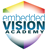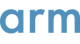# ArmThis chapter describes an example optimization process for creating image pyramids.This chapter describes the theory of image pyramid creation.This chapter introduces OpenCL, pyramid, and the suitability of pyramid for GPU compute.This chapter describes how to re-use the sample implementation and test the performance of optimizations.This chapter describes the requirements to run this sample and the example test platform which generates the results that this guide shows.This chapter describes some conclusions from the optimization process.This chapter describes some further optimizations to the kernel.This chapter describes an example implementation of Canny edge detection.This chapter describes the theory of Canny edge detection.Canny edge detection is a popular, tunable algorithm that extracts high-quality edges from images.